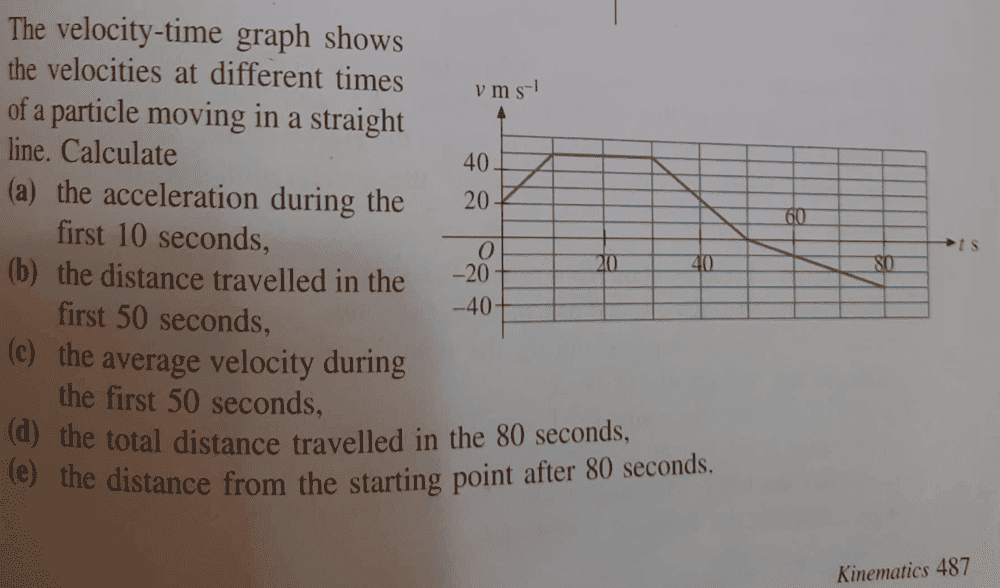# Find the distance traveled on the velocity-time graph

Gold Member
Homework Statement:
part b. find the distance travelled in the first ##50## seconds
Relevant Equations:
kinematicsnow this is my approach, my interest in on part b
distance travelled= area under the graph between time ##t=0## and ##t=50##,
area = ##0.5(40+20)10+ (20 × 40) + 0.5(40+20)10+ 0.5×10×20##
Area= ##300+800+300+100=1500m##
now textbook says i am wrong, where have i made a mistake?